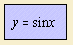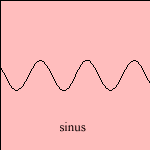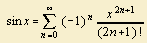# sine

## trigonometricIn the antiquity the sine was used as the length of a chord. Given a circle with a certain radius, for a large amount of values of the angles the lengths of chords were written down 1). Such cord tables can be found in Ptolemy's' Almagest (150 AC) and in the Indian Surya Siddanta, and were used especially by astronomers.
In the late Middle Ages there was a short period that one used to write the tangent as sixtieths of the radius, and sixtieths of sixtieths. It was the German astronomer Regiomontanus (Johannes Muller, c. 1450), who prevented this practice. He wrote the trigonometric functions as lengths of chords for a circle with a radius of 10n (n = 5 - 15), so that the results could be written as whole numbers.

It was not earlier than the 19th century that the sine got his actual meaning as the proportion of line segments.
Geometrically the sine can be defined, in a rectangular triangle (Euler) as the proportion of the opposite side to the hypotenuse. Another way to define the curve is as a power series 2).
A sine curve is also called a sinusoid.

A point moves with constant speed on the circumference of a circle. Take as center of the circle the origin, then the coordinates of the moving point as function of the rolled angle a are: (cos(a), sin(a)). So the component along an arbitrary diameter is a sine function of time. This is the definition of a harmonic oscillation. Necessary for this movement is a force, which is proportional to the movement: F = - k x.
The sine obeys this rule, because for its second derivative applies: y'' = - y.

In fact, the harmonic oscillation is the form in which we meet the sine in our physical world.
A moving harmonic oscillation gives as result a sinusoid wave. The periodicity of the sine is called the wavelength, the amplitude is the factor of sin x.
Imagine a triangle, the sine formula states that the ratio of the sine of an angle and the opposite side is equal for all three angles.
An equalized sine is the result when the negative values have been turned: y = |sin x|.

Sine means bend, curve, bosom. It is a literal translation of the Arab gaib, what has been derived from gib, a way to spell the Indian jya (cord). So there is a relation with the old habit of using the sine as the length of a cord. In natural life we see the sine form in the bosom of a wife.
In screwing activities we make a three dimensional helical line, when projecting the line on a surface through the screw's axis the result is again a sine.
In the bicycle-loving country of Holland, research led to the conclusion that a street threshold (to limit the speed of the cars) in the form of a sine is the most comfortable form for bicycling.

The cosine is defined - in the same rectangular triangle - as the proportion of the adjacent side to the hypotenuse. The cosine is a translated sine: cos x = sin (x + π/2).
The cosine formula is an extension of the theorem of Pythagoras. For a triangle without a rectangular angle, it states that a2 = b2 + c2 - 2bc cosφ3).

Derived from the sine are some functions, that have been used in older times:
- the versine or versed sine is defined as: versin(x) or vers(x) = 1 - cos(x)
- the vercosine or versed cosine is defined as: vercosin(x) = 1 + cos(x)
- the coversine or coversed sine is defined as: coversin(x) = 1 - sin(x)
- the covercosine or coversed cosine is defined as: covercosin(x) = 1 + sin(x)
- the haversine or haversed sine is defined as haversin(x) or hav(x) = 1/2 versin(x)
- the havercosine or haversed cosine is defined as havercosin(x) = 1/2 vercosin(x)
- the hacoversine or cohaversine or hacoversed sine is defined as hacoversin(x) = 1/2 coversin(x)
- the hacovercosine or cohavercosine or hacoversed cosine is defined as hacovercosin(x) = 1/2 covercosin(x)

The inverse functions of the sine and the cosine are called the arc sine arcsin(x) and the arc cosine arccos(x), respectively. These are cyclometric functions.

Sine series and cosine series are obtained while adding sines or cosines, in the sine summation.

notes

1) The length of a cord can be written in our current notation as: cord(a) = 2 R sin (a/2)

2) The sine as a power series:3) The angle π is the angle opposite line a.